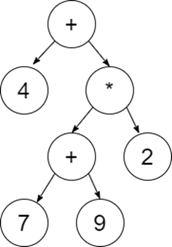# Python Program to Construct an Expression Tree of a given Expression

Expression trees are those in which the leaf nodes have the values to be operated, and the internal nodes contain the operator on which the leaf node will be performed.

Example: 4 + ((7 + 9) * 2) will have an expression tree like -## Approach to solve this Problem

In order to construct an Expression Tree for a given expression, we generally use Stack Data Structure. Initially we Iterate over the given postfix expression and follow the steps as given below -

• If we get an operand in the given expression, then push it in the stack. It will become the root of the expression Tree.
• If an operator gets two values in the expression, then add in the expression tree as its child, and push them in the current node.
• Repeat Step-1 and Step-2 until we don't complete over our given expression.

## Example

Live Demo

class stack:
def __init__(self):
self.arr = []
def push(self, data):
self.arr.append(data)
def pop(self):
try:
return self.arr.pop(-1)
except:
pass
def top(self):
try:
return self.arr[-1]
except:
pass
def size(self):
return len(self.arr)
# node class for expression tree
class node:
def __init__(self, data):
self.data = data
self.left = None
self.right = None
# expression tree class
class exp_tree:
def __init__(self, postfix_exp):
self.exp = postfix_exp
self.root = None
self.createTree(self.exp)
def isOperator(self, char):
optr = [" ", "-", "*", "/", "^"]
if char in optr: # if given char is operator
return True # then return true
return False # else return false
def createTree(self, exp):
s = stack()
# store those operator node whose any child node is NULL
self.root = node(exp[-1])
# last character of postfix expression is always an operator
s.push(self.root)
# travel on rest of the postfix expression
for i in "".join(reversed(exp[:-1])):
curr_node = s.top()
if not curr_node.right:
# if right node of current node is NULL
temp = node(i)
curr_node.right = temp
if self.isOperator(i):
s.push(temp)
else: # if left node of current node is NULL
temp = node(i)
curr_node.left = temp
# if no child node of current node is NULL
s.pop() # pop current from stack
if self.isOperator(i):
s.push(temp)
# inorder traversal of expression tree
# inorder traversal = > left, root, right
def infixExp(self):
# inorder traversal of expression tree give infix expression
self.inorder(self.root)
print()
if __name__ == "__main__":
postfixExp = "ab ef*g*-"
et = exp_tree(postfixExp)
et.infixExp()

Running the above code will generate the output as,

## Output

(a + b - e * f * g)

Explanation:

Constructing a tree from a given expression will generate the output such that the operand will become the root of the node and the rest of the numbers will become the child nodes of the expression tree.